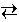# Chemical Equilibria

## Le Châtelier's Principle: Effect of a Change in Temperature

### Concepts

In a previous experiment you saw how Le Châtelier's Principle can be used to predict how the equilibrium amounts of material in a system are affected by the addition or removal of a reactant or product. This principle, stated below, applies to other types of stresses as well.

When a stress is brought to bear on a system at equilibrium, the system will react in the direction that serves to relieve the stress.

A change in the analytical amount of a reactant or product produces a stoichiometric stress on the system. A change in temperature also constitutes a stress on the system, but what is the nature of this stress? Unlike changes in the analytical amounts of reactants and products, a change in temperature produces a change in the equilibrium constant itself. To understand the effect of a temperature change on the value of the equilibrium constant, it is necessary to understand how temperature affects a reaction.

An increase in temperature is the result of the flow of heat into the system. Conversely a flow of heat out of the system reduces the temperature. A reaction itself can be a source or sink for heat. The heat flow for a reaction is characterized by the Enthalpy of Reaction or the Heat of Reaction, ΔHrxn.

If ΔHrxn > 0, the reaction is said to be endothermic, which means that the reaction draws heat from its surroundings as the reaction occurs.

If ΔHrxn < 0, the reaction is said to be exothermic, which means that the reaction releases heat into its surroundings as the reaction occurs.

Suppose an exothermic reaction is at equilibrium, and then the temperature of the system is increased. The increase in temperature corresponds to introducing heat into the system. This influx of heat pushes the system away from equilibrium and nature restores equilibrium by removing some of this additional heat. Because the reaction is exothermic, the reaction produces heat when it proceeds in the forward direction. Le Châtelier's Principle states that the system will react to remove the added heat, thus the reaction must proceed in the reverse direction, converting products back to reactants. Conversely if the temperature of the system were decreased (heat removed from the system), the system would react in a direction that opposed the removal of the heat. The forward reaction would thus occur to release heat in an attempt to offset the heat that was removed from the system.

Bear in mind that the system is being held at a specific temperature, so the net reaction (which may produce or release heat) does not actually change the temperature of the system. That extra heat is removed or replaced as necessary to maintain the temperature.

The steam reforming reaction studied in the previous experiment is an example of an endothermic reaction.

C (s) + H2O (g)CO (g) + H2 (g)

ΔHrxn = + 131.3 kJ mole-1

As an endothermic reaction, the steam reforming reaction draws heat out of the system as it occurs. Although it is not strictly correct to include heat in a chemical equation, which shows stoichiometric relations between physical substances, one could loosely represent the endothermic nature of the reaction by writing heat as a reactant:

heat + C (s) + H2O (g)CO (g) + H2 (g)

The advantage of this construct is that it permits the appplication of Le Châtelier's Principle through the same reasoning as employed when the analytical amount of a substance is changed.

The temperature-dependence of the equilibrium constant, KP in this case, is determined by the enthalpy of reaction.

### Experiment

Objective:

Explore the effect of a change in temperature on the equilibrium composition of a system.

Experiment:

Once again the steam reforming reaction is used to illustrate Le Châtelier's Principle. In this case the effect of a change in temperature will be examined. The reset button resets the system temperature to 1000. K. Use the slider to dynamically change the temperature of the system and carefully observe the effect of the temperature change on the equilibrium amount of each reactant and each product.

1. Reset the experiment. Use the slider to increase the temperature of the system. What happens to the equilibrium amounts of hydrogen and carbon monoxide in the system? What happens to the equilibrium amounts of carbon and water? Is this behavior consistent with Le Châtelier's Principle?

2. Reset the experiment. Use the equilibrium amounts of each species, the system volume of 10.0 L, and the system temperature of 1000. K to calculate the equilibrium constant KP. Change the system temperature to 1200. K. What effect should this change in temperature have on the equilibrium constant? Check your prediction by using the equilibrium amounts to calculate KP at 2000. K. Repeat this analysis at 800. K.

 Temperature K Equilibrium Amounts of Material in the Glass Bulb C moles           H2O moles           CO moles           H2 molesLe Châtelier's Principle: Changes in VolumeChemical Equilibria Home PageVirtual Chemistry Home Page

Temperature.html version 2.1